Adapted for the Internet from:Why God Doesn't Exist
 Particles  cannot model(c = ƒ λ)

1.0   The ridiculous notion of frequency of Mathematics

The mathematicians define frequency and wavelength respectively as:

Frequency is a measure of the number of occurrences of a repeating event per
unit time.

wavelength is the distance between repeating units of a propagating wave of
a given frequency. 

From these definitions, the mechanics somehow conclude that:

“ Frequency has an inverse relationship to the concept of wavelength,simply,
frequency is inversely proportional to wavelength λ. .” 

“ in order to make the equation still correct, an increase in frequency results in a
decrease in wavelength… Lower frequencies have longer wavelengths.” 

This means that if we increase a wave’s frequency, its wavelength decreases and vice versa pursuant to the
relation (ƒ = c / λ),  where ƒ = frequency,  c = velocity of light, and λ= wavelength.

However, this is not true. The mathematicians are misleading you when they make such claims. In QM,
frequency does not always increase at the expense of wavelength. When light crosses from air to water, for
example, the mechanics hold frequency constant and decrease both the wavelength and the velocity of light. 

“ When waves travel from one medium to another, their frequency remains exactly
the same — only their wave-length and/or speed changes.” 

Under this scenario, the wavelength is shorter, the frequency remains the same, and little c (velocity or speed of
light)  decreases. Thus, wavelength and frequency do not have an inverse relationship as initially advertised
(Fig. 1). The mathematicians live with these inconsistencies and prod on like fools without ever resolving them.

The reason behind these contradictory statements is that the mathematicians define frequency in terms of time
and wavelength in terms of length. They measure one with a clock and the other with a ruler or caliper. Of course,
if we assume that light has different speeds and, additionally, factor in relativity’s warped space, light never has a
chance to travel rectilinearly, let alone at a constant speed. Yet the mathematicians always use little c in all of their
relativity and quantum calculations!
 Fig. 1   Quantum's ridiculous version of frequency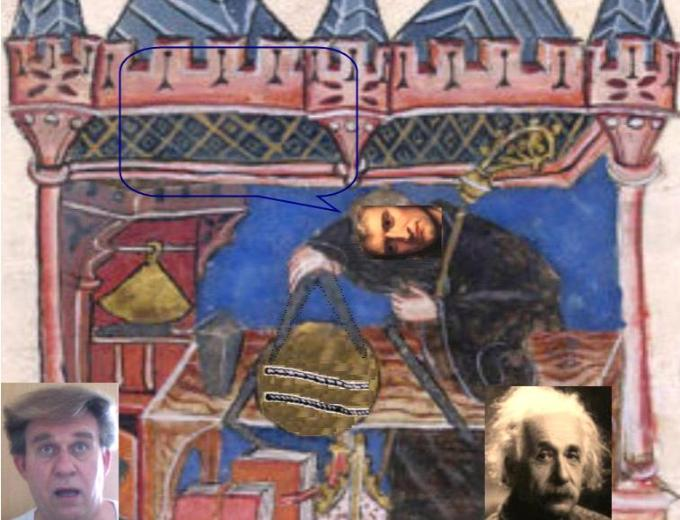I don't understand it, Al!     I can't get the size of the link to be constant! When I twist these ropes, the number of links increases, but then each link becomes shorter!
 The morons  of  Mathematical  Physics  do frequency the way  of Ptolemy. They hold frequency constant and believe that light travels at different speeds through different media (a phenomenon known as refractive index). The ridiculous 'refractive index' version of frequency is not a reality of nature, but merely a convention. QM’s Ptolemaic explanation owes its livelihood to the amusing fact that the mathematicianshave defined frequency in terms of time and wavelength in terms of length. In QM, the speed of light is variable, but the mathematicians use it in all of their equations as a constant (i.e., little c)!It's probably some particle going from one end to the other. You have a pair of bad ropes, Newt!  You should buy new ones.
 For example, the mathematicians at the University of Riverside's  Physics FAQ have light traveling at different speeds through different media, constant for SR, and variable for GR. Then for unexplained reasons they tell you that "the photon can never be at rest"Why not you stupid morons? If you can slow a photon down and explain that it sometimes crashes against an electron, what physical reason prevents the photon from grinding down to a standstill?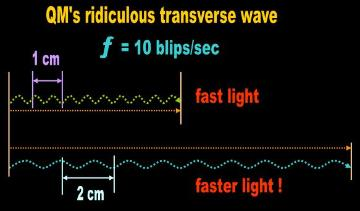3.0        In QM, photons accelerate for no reason

A more vexing question that QM’s varying speed of light raises is ‘What causes light to suddenly accelerate
when it crosses the boundary to the less dense medium after refracting?’ For instance, when light crosses
from air to glass, the mathematicians claim that the speed of light slowed down from about 300,000 to
220,000 km per second. They explain that the reduction in the speed of light is solely due to a decrease in
wavelength. Frequency (blips per second) remains the same:

" When visible light in air enters a medium such as glass, the velocity of light
decreases to 75% of its velocity in air... As the light enters the glass the
wavelength changes to a smaller value λ'." 

" its wavelength increases or decreases but its frequency remains constant." 

The question now is 'What physical mechanism accelerates a photon from 200,000 km/sec to 300,000 km/sec
when it crosses again from glass to air (Fig. 6)? We cannot explain this acceleration with particles. So much
for the particle model of light. The wave theorists think they have an answer:

" The waves are continuous and remain connected as they pass from one
medium to another. We can think of it like a long line of people running into
the ocean. As the first few people run into the water, they're slowed down
because it's harder to run in water. Thus, they bunch up and stay bunched up
as they run through the water. When everyone in the line has entered the water,
we would see a line of people all running in the same direction, but the line
would be shorter and the people would be bunched close together. If they run
back to the beach, the first few people would clear the water and run faster.
Eventually everyone will have cleared the water and would be running at the
original pace with the original spacing between persons." 

" A useful analogy in explaining the refraction of light would be to imagine a
marching band as they march from pavement (a fast medium) into mud (a
slower medium) The marchers on the side that runs into the mud first will
slow down first. This causes the whole band to pivot slightly toward the
normal (make a smaller angle from the normal)." 

These analogies are absolutely stunning and show just how ignorant our mathematicians are! They are saying
that the right hand side of the wave front (without regard to the individual EM waves) waits up for the left at the
air-glass interface. Then they march together in a straight line through the glass. When they front reaches the
glass-air interface, the left side of the front emerges first and again has a head start in speed over the right hand
side.

Actually, the left hand side of the beam would have an advantage over the right hand side of the beam
throughout its itinerary in the glass. The analogy 'predicts' that the wavefront will be warped during its entire
trajectory in the glass. The left hand side will encounter the denser glass first, but it will continue to have an
advantage over the right hand side which simply experiences the denser medium later. Therefore, if we allow
the mathematicians to get away with this explanation (which is the only physical interpretation that
Mathematical Physics has for refraction), we should observe that the wavefront from the Sun sweeping
through the thick layer of air in which we live should always be warped! After 3000 years of research the
stupid idiots of Mathematics are not even able to explain a simple phenomenon such as refraction!
 Fig. 6   The speed of light

A rope does not run into these predicaments. It is a continuous entity that doesn’t travel through a medium as
waves or particles are alleged to do. An EM rope interconnects any two atoms in the Universe. Thus, a torque
signal propagates from an atom in the Sun to an atom comprising the Earth's atmosphere (Fig. 7). This atom in
turn relays the signal to an atom comprising the ocean which relays the signal to an atom elsewhere  (Fig. 8). In
Science and in Physics, both frequency and wavelength are defined in terms of length. Frequency is simply the
number of links per unit length of rope. If we increase the number of links per meter (increase in frequency), we
necessarily end up with smaller links (decrease in wavelength). The rope has different link lengths (as opposed
to wavelengths) in each medium. The velocity of light along the rope remains constant irrespective of the medium
the signal traverses!
 The idiots of Mathematics don’t measure the speed of light. They calculate angles. Light travels at 300,000 km/sec in a vacuum. The mechanics tell you that it slows down to around 220,000 km/sec when it penetrates into glass. No problem! What is it that accelerates light back to 300,000 km/sec when the photon leaves the glass and returns to the vacuum? Newton’s 3rd Law requires a force contributed by an extrinsic agent. Einstein’s relativity requires space curvature to deflect the path of light. What's it gonna be?
 Fig. 2   Only a rope configuration explains why (ƒ = c / λ).
 Rope ‘frequency’: links per unit length
 Take a rope and torque it a few times. You will verify that you now have shorter links and more links per unit length. In Physics, frequency is the number of links per meter. Hence, if we increase the length of each link, we can fit fewer links fora given length of rope. We're done! The rope requires that the velocity of light be a constant because frequency is a function of unit length.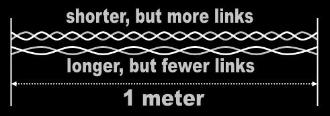2.0   Particle frequency?

How does a single traveling particle simulate frequency, wavelength, and amplitude anyways? I guess this
question tasks the imagination of anyone attempting it with one particle. A single particle can go in any
direction…but not simultaneously (Fig. 4)! While it goes up, it can’t also go down and forward. The down and
forward motions necessarily occupy different frames in the Universal Movie  (Fig. 4 B). Therefore, the
mathematicians are going to have to talk about behavior of a stream of traveling particles. They can't just do it
with one.
 Fig. 4   The footprints that the mathematicians make in space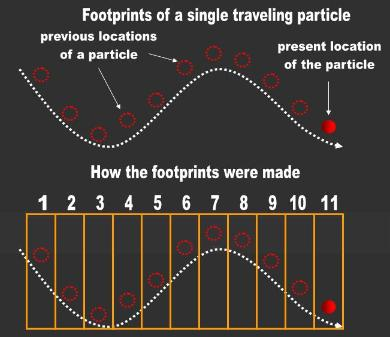A particle can perhaps generate a series of footprints in the sand, but not in space. When the mathematician draws a sine wave, he draws all the points on thesame page. When he is finished, you can see all the points simultaneously like you would see the footprints you left while walking on the beach. You cannot visualize a 'wave' in space as if staring ata photograph if the wave consists of a single particle moving forward. Each location of the particle has now vanished. It is now in the past. Motion requires memory of an object's previous location. Motion is not a photograph, but a movie of a moving object. A wave made by a single particle is not a standalone trace
 Fg. 5
 The particle mathematicians have no explanations. In fact, Newton's corpuscle theory 'predicted' that light would travel faster through denser media. So much for the particle model of light! It dies here!The wave mathematicians claim that a wave encounters more resistance entering and less leavingthe glass. They invoke the analogy of a marching band which finds the marchers on one side waiting for the marchers on the other. The theorists illustrate this analogy with a straight wavefront.Actually, the analogy is misleading. Under this scenario, one side of the wavefront simply enters the medium before the other and begins to travel slower. The other side has no chance of ever catching up. The entire wavefront is curved as portions enter the glass at different times. The side that entered first also emerges first at the glass-air interface. Now the front should curve in the opposite directionif this analogy has any merit. Therefore, according to the mathematicians, a denser medium should transform wavefronts from concave to convex. In fact, light should never have a straight wavefrontin any medium except the vacuum. The mathematicians' analogy and official explanation is absolute bullshit! It has no merits whatsoever because it violates logic and observation!Again, the root of the problem with this ‘acceleration’ version of light is that the mathematicians relyon a misconceived definition of frequency. They hold frequency constant and decrease both wavelength and the speed of light through a denser medium. The reason for this ridiculousconclusion has its origin in that the mathematicians have defined frequency in terms of time and wavelength in terms of 'length' (i.e., space). The speed of light rules out both particles and waves. These models cannot simulate observation.

With the rope model, the velocity of light equation (ƒ = c / λ) holds true in every situation. Light always has the
same speed irrespective of medium. In Thread Theory, frequency and wavelength are defined in terms of length.
Take two strings, wind them around each other, and torque them until you have 10 links when your hands are 1
meter apart. The only way you can double the number of links or waves for the same spread is if you coil or
torque the string some more (Fig. 2). In fact, with this simple experiment, you will verify the reason the signal of
light always travels at the same speed irrespective of medium.

So now the mathematician proposes that frequency refers to the separation between two or more particles
in a stream: the lower the distance between two particles, the higher the frequency (Fig. 5).

" The waves are continuous and remain connected as they pass from one medium
to another. We can think of it like a long line of people running into the ocean." 

Under this scenario, the particle does not move forward, but rather jumps up and down in place. They call it
the standing wave model of light.

Fine! Now how do we explain amplitude with this model if amplitude runs perpendicular to frequency? For
instance, if we increase the amplitude or the frequency, we necessarily drew more particles into a given length
of wave. This example necessarily requires that particles also move forward. Nevertheless, the mathematicians
define frequency as the number of blips per second (time) and not as the distance between two different particles
each going up and down in the same region of space. But assuming the mathematicians insist on their standing
wave model of light, does this mean that the wave extends uninterruptedly all the way from the Andromeda
Galaxy two million light years away to an atom here on Earth? Recall that EM waves originate in atoms and are
absorbed by atoms. If a wave is not interrupted by anything from Andromeda to Earth, the wave is necessarily
two million light years long! A standing wave may also be generated with two anti-parallel traveling waves. So,
is this 'thing' shaking up and down in situ all along this humongous distance?

So the mathematician changes his tune again in order to avert disaster. He now proposes that light consists of
a stream of particles each of which travels sinusoidally: up and down as well as forward. Under this scenario
we are not talking about a standing wave, but about a traveling wave. At face value, this model appear to be
consistent with frequency. The location occupied by one particle a second ago is now taken over by another
one in the group. But keep in mind that if this wave arrives uninterruptedly from Andromeda, it is also two
million light years long. If it comes from the other side of the Universe, its length is simply unimaginable.

However the traveling wave has even more insurmountable conceptual problems. The most obvious one is that
each particle comprising the wave must travel faster than the overall wave. The wave just travels forward. The
particle within the wave must in addition go up and down. In fact, if we increase the amplitude, the particles
comprising the wave now have to travel even longer north-south distances in the same amount of time. Certainly,
the beam of light has no patience to wait for any particular component.

Of course, the idiots of Mathematics noticed the problem. (They are not THAT stupid!) So they had to develop
some kind of loophole for when an elementary school kid pointed out this discrepancy to them:

" superluminal communication is not possible in this case, since the signal velocity
remains less than the speed of light." 

" The phase velocity of electromagnetic radiation may under certain circumstances...
exceed the speed of light in a vacuum, but this does not indicate any superluminal
information or energy transfer." 

" the phase velocity of a wave corresponds to the propagation speed of a theoretical
single-frequency (purely monochromatic) component of the wave at that frequency.
Such a wave component must be infinite in extent and of constant amplitude
(otherwise it is not truly monochromatic), and so cannot convey any information...
a phase velocity above c does not imply the propagation of signals with a velocity
above c...  The group velocity of a wave (e.g. a light beam) may also exceed c in some
circumstances. In such cases... the maximum of the envelope of a pulse may travel
with a velocity above c. However, even this situation does not imply the propagation
of signals with a velocity above c" 

" the phase velocity is yet another example of a speed faster than light which cannot
carry a message." 

Signals? Information? Messages? Who said anything about signals and information? We are talking about a
particle which the idiots of Quantum say is a component of a wave. (The alternative is to say that an EM wave is
devoid of a medium (i.e., that energy is absolutely nothing)! The stupid idiot of mathematics thinks he answered
the question by saying that the overall beam travels at the same rate, that the 'signal' or 'information' reaches
the observer at the scheduled time irrespective of what particles do within the beam. That's not the question.
Neither does the question have anything to do with what an extrinsic mathematician observes or measures.
The question is whether a particle within the beam  travels faster than light. This is exclusively a conceptual
issue. If the photon, wave-packet, wave, state vector, or whatever the mathematicians want to call their
contraption travels at 300,000 km per second, a particle within the photon, wave-packet, or wave must
necessarily travel faster than 300,000 km per second if it is going to oscillate up and down! Period! This defeats
the perpetual claim of Special Relativity which says that nothing, not even a particle within a wave, can travel
faster than a photon because it will require 'infinite energy' (whatever that is)! Relativists and mechanics have
a logical contradiction! Then they have the gall to boast that:

" There is nothing uncertain about the speed of light (c)." (p. 577) 

The bottom line is that the morons of relativity have not resolved, will never resolve, and have no interest in
resolving whether anything can travel faster than light:

" This makes it very difficult, perhaps impossible, to define global FTL unambiguously...
If you were expecting me to finish this section with a precise definition of what is
meant by FTL travel and FTL communication I am afraid I have to disappoint you.
The above difficulties are insurmountable." 

So after a two hour talk, the expert tells you that he has no idea what he's talking about.  Absolutely stunning!

The traveling wave also has two insurmountable frequency problems
Frequency is a measure of how compressed a series of wavelengths are.
Mathematical frequency is the number of wavelengths per second. If we
increase the frequency or the amplitude, we necessarily created more particles.
With the traveling wave is that now the mathematician has to measure from
where the particle was before to where it is now within the beam. If we increase
the amplitude, the particle has to travel a longer distance, and the mathematician
ends up increasing the frequency. This contradicts what we observe. Increasing
the amplitude of a wave does not increase its frequency.

Therefore, under any version the mathematicians choose, we cannot reconcile frequency with particles. From a
strictly conceptual perspective a wave cannot be comprised of particles.
 The mathematicians define frequency in terms of time. The frequency and wave of Mathematics are inherently dynamic. If light consists of astanding wave, this means that this medium oscillates up and down in in place. How can the mathematicians reconcile their definition of frequency with this proposal if frequency is the distance between two locations, meaning that the particle moved forward. (e.g., the difference between 450 and 460 nm wavelength would require the particle to changelocation).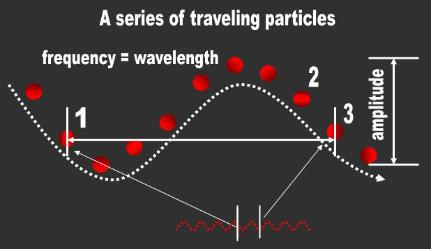A
 B
 If, instead, the mathematicians are saying that light consists of two anti-parallel traveling waves, each particle comprising the waves must travel up and down AS WELL AS forward. Does the electric field travel in the opposite direction to the magnetic field? Do the particles that constitute each field collide? How do we define frequency if the particle of the electric field is going in opposite direction to the one constituting the magnetic field? Do these EM fields extend uninterruptedly from the Andromeda Galaxy to us? Is the magnetic field generated on Earth whereas the Electric field is generated at Andromeda? The questions are endless and have no resolution under the hypothesis that a wave is made of particles. We simply cannot reconcile frequency as defined in Mathematics with a wave made of particles.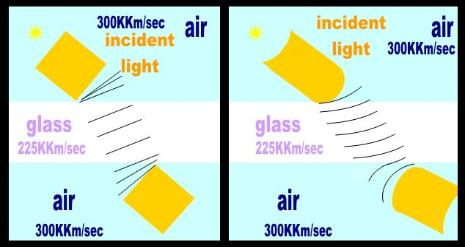Every atom in the Sun is connected via an EM rope to every atom on Earth, Including everyatom in your body. This is not a one to one correspondence. It is an all to all correspondence. Light consists of torque signals propagatingalong these ropes. The Sun swings the Earth around like scores of Lilliputians would swirl Gulliver around at the end of gazillions ofthreads.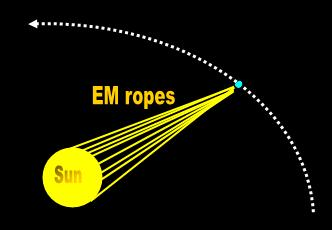Fig. 7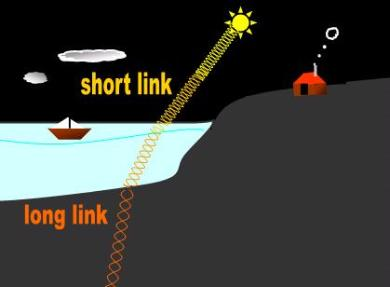Fig. 8
 An atom 'relays' a torque signal to another through the rope that interconnects them. Thus, an atom in the Sun sends the signal to an atom in the atmosphere which relays the signal to an atom comprising the ocean which relays it to an atom in the center of the Earth. (The atom in the Sun is also connected directly to these same atoms.)  Link lengths (wavelength) increase andthe number of links per unit length (frequency) decreases in denser media. In Physics, 'frequency' is always inversely proportional to 'wavelength' and thevelocity of the torque signal is constant.
 The mechanics should have figured this out decades ago. Instead, they kept modeling and simulating their equations with particles. Not being particularly keen on architecture, the mathematicians focused on the signal going back and forth and neglected the intermediary.They never progressed beyond the particle since they crowned  Newt king!
 Fig. 3
 If, as QM insinuates, a wave of light is made of moving particles, an increase in the amplitude would entail either(A) more particles for higher peaks or (B) that each particle have a longer itinerary: the higher the amplitude, the longer the path a given particle that comprises the wave must travel. The sigmoidal or S-shaped path traveledby each particle implies that they propagate faster than light. (The littlec is contingent on rectilinear motion.)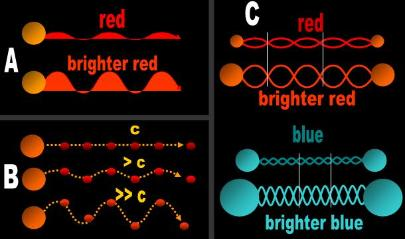The questions you must ask yourself are: What is it that deflects a particle from its rectilinear path? If the particles oscillate up and down in situ, what is it that moves forward in this scheme? Is a ‘photon’ (wave-packet) a standing or a traveling wave?Under the rope hypothesis (C), amplitude is simply a taller link. This phenomenon is independent of frequency. Red may have the same amplitude as blue despite that both have different link-lengths. A rope explains why amplitude is detected as intensity.

2.0   Amplitude

Particles also have a very difficult time simulating amplitude increases. What extrinsic agent would cause a
particle to go higher and lower? If a photon travels forward at c within a given medium as well sinusoidally
(i.e., if it must go up and down as well as forward), it must necessarily travel faster than light and faster still
during amplitude increases. Yet relativity claims that the entire beam of light always travels at the same speed.

The mathematician thinks he has an answer. Perhaps the particle comprising the beam of light only moves
up and down while the ‘wave’ passes through. This concept is known as a standing wave. Gazillions of
particles, induced by evil spirits, move up and down in a long region of space. Think of a rope tied to a pole
that you swing up and down. Any portion of the rope that you analyze merely moves in place.

Unfortunately, this explanation won’t cut it because the photon of Mathematical Physics is supposed to be a
traveling wave. The mathematical photon ball moves forward as well as up and down.
But assuming that a corpuscle comprising a beam of light only moves up and down while the ghost of motion
passes through, this scenario runs up against two insurmountable conceptual problems:

1.      The proponent would have to justify what causes each particle to move up and down
in the first place.

2.      The alleged wave is strictly an observer-related phenomenon. Without an observer,
there is no wave. All we have is each particle moving up and down.

To show just how trivial wave dynamics are without an observer, assume that each particle only moves up and
down. How does light cause pressure on a surface (i.e., in the ‘forward’ direction) at the other end as
demonstrated by Hertz and Lebedev? Or how is the photon generated inside the atom to begin with if light
simply consists of a string of particles moving strictly up and down between two atoms?

On the other hand, a rope has no problems simulating amplitude. Take a rope and you will note that each link
already has built-in amplitude. When the amplitude increases, the thread that makes up a link does not stretch.
A thread is not like a rubber band. A rope reels in more thread to attain higher amplitude      (Fig. 3). Thus, an
increase in amplitude does not entail a faster signal. The rope continues torquing at its regular rate. Matter is
not created out of nothing as it is in Mathematical Physics.

c
Compare

How light works according to the mathematicians of Quantum Mechanics:

 The animated rope version of lightClick on the image if you wish to see two atoms sending torque waves (light) to each other while they 'Quantum Jump'...in slow motion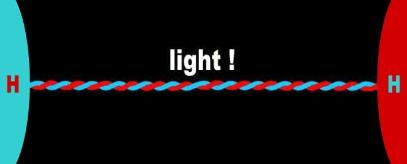________________________________________________________________________________________